Function Repository Resource:

# IntegerPartitionFrequency

Counts the number of times an integer k appears within all possible ways to partition an integer n without calculating n’s integer partitions

Contributed by: Edmund B Robinson
 ResourceFunction["IntegerPartitionFrequency"][n,k] gives the number of times k appears within all possible ways to partition n into smaller integers.

## Details and Options

ResourceFunction["IntegerPartitionFrequency"][n,k] requires positive integers n and k such that nk.
ResourceFunction["IntegerPartitionFrequency"][n,k] does not compute the integer partitions of n in its calculation.
ResourceFunction["IntegerPartitionFrequency"] makes use of an integer’s partitions being combinations instead of permutations. It calculates the number of partitions {k1,} with k in position 1 (leaving n-k to partition), then {k1,k2,} with k in positions 1 and 2 (leaving n-2k to partition), and so on up to {k1,k2,,kj,} for njk0. Then, it totals these counts.

## Examples

### Basic Examples (2)

Calculate how many times the integers 20 to 25 appear in all possible integer partitions of 100:

 In:=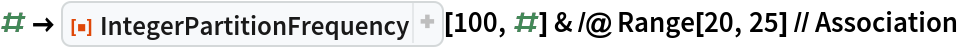Out=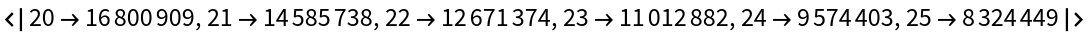Compute how many times the integer 19 appears in all possible integer partitions of 500:

 In:=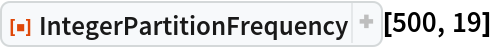Out=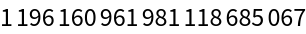### Scope (2)

IntegerPartitionFrequency calculates its count orders of magnitude faster than calculating the partitions of n and then counting the occurrences of k within the partitions. For example, the number of times 1 appears in all possible integer partitions of 75.

Generate partitions and count:

 In:=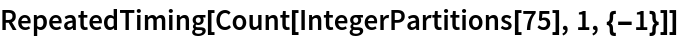Out=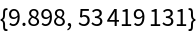Count by IntegerPartitionFrequency:

 In:=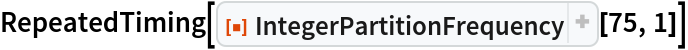Out=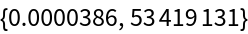### Neat Examples (1)

Plot how many times each integer 1,2,,100 appears in all possible integer partitions of 100:

 In:=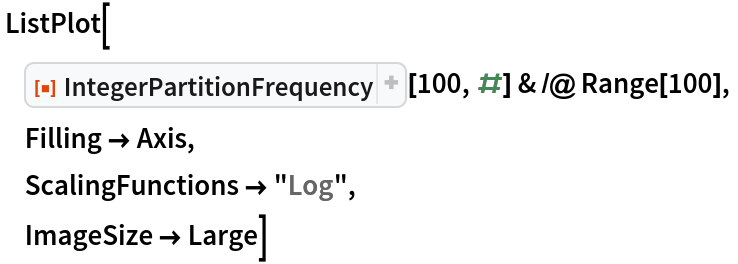Out=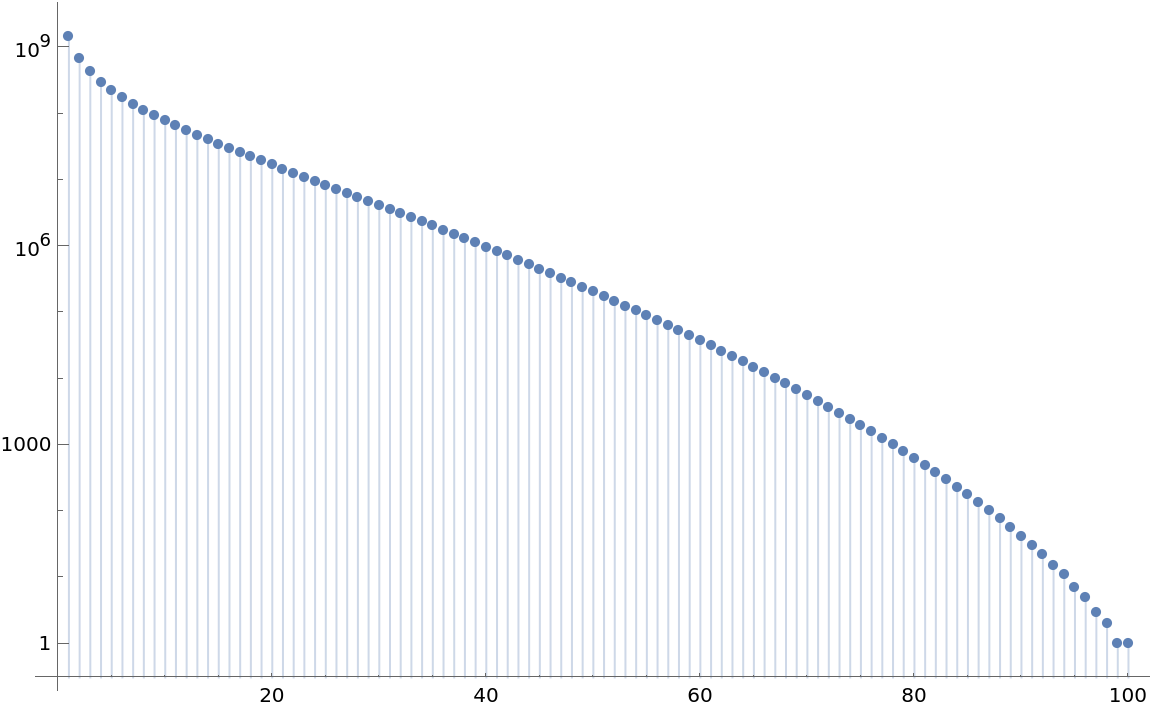## Publisher

Edmund B Robinson

## Version History

• 1.0.0 – 10 October 2019